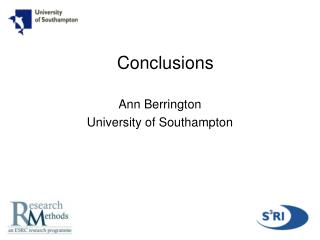DownloadDownload PresentationConclusions

Conclusions

Télécharger la présentationConclusions

- - - - - - - - - - - - - - - - - - - - - - - - - - - E N D - - - - - - - - - - - - - - - - - - - - - - - - - - -
Presentation Transcript

1. Conclusions Ann Berrington University of Southampton

2. Review of this morning’s aims • Aim: • To describe, illustrate, compare and contrast the use of: • graphical chain models • structural equation models • random effect models • for the analysis of panel data • What are the advantages and disadvantages? • How different are they? – convergences and recent developments

3. Advantages and disadvantages of graphical chain models • Advantages • Fit relatively simple models for the various types of response • Can use standard methods to handle the complex survey design and non-response • Can use Markov properties to draw conclusions about conditional independence and dependence structure of subsets of variables • Disadvantages • Not using all the information in the repeated measures or modelling the reciprocal relationship simultaneously • Difficult to adjust for measurement error • Difficult to assess overall goodness of fit of the model or to test for equality of effects across time

4. Advantages and disadvantages of random effects models (MLM) • Advantages • Easy to expand to 3 or more levels • Can explicitly incorporate alternative link functions e.g. for binary and nominal outcomes • Uses data available – e.g. can include individuals until they drop out • Disadvantages • Assumption of independence between the random effects and the fixed effects • Random intercept (but not random coefficient models) assume exchangeable correlation structure

5. Advantages and disadvantages of structural equation models (SEM) • Advantages • Provides an overall measure of model fit • Can include multiple indicator latent variables • Can decompose total effects into direct and indirect effects • Disadvantages • Difficult to incorporate 3+ levels • Requires careful interpretation of parameters • Many packages do not allow for inclusion of weights, complex survey design • Some packages do not allow for categorical dependent variables esp. those with more than two categories

6. Convergence in methods • Latent variables could be incorporated to some extent in graphical models (e.g. could have used factor loadings for items used for gender role attitude to create a new variable) • 2-level random effects MLM growth model is analytically equivalent to a SEM estimation • In MLM time is entered as a predictor variable • In SEM time is entered as the factor loadings relating the repeated measures to the underlying latent factors

7. Recent developments include: • All approaches - methods for incorporation of weights and complex survey design • MLM – models for simultaneous processes • Extension of SEM to 3+ levels • GLLAMM - generalized linear latent and multilevel models • MPlus – general model framework – e.g. incorporation of categorical dependent variables using polychoric correlations and weighted least squares estimation

8. Result from Random Intercept Model Random-effects ML regression Number of obs = 5716 Group variable (i): pid Number of groups = 1429 Random effects u_i ~ Gaussian Obs per group: min = 4 avg = 4.0 max = 4 LR chi2(1) = 80.17 Log likelihood = -14015.718 Prob > chi2 = 0.0000 ------------------------------------------------------------------------------ score | Coef. Std. Err. z P>|z| [95% Conf. Interval] -------------+---------------------------------------------------------------- time | -.1162701 .0129252 -9.00 0.000 -.1416031 -.0909371 _cons | 20.37418 .0880119 231.49 0.000 20.20168 20.54668 -------------+---------------------------------------------------------------- /sigma_u | 2.779735 .0602076 46.17 0.000 2.66173 2.89774 /sigma_e | 2.185095 .0235981 92.60 0.000 2.138844 2.231347 -------------+---------------------------------------------------------------- rho | .6180766 .0117726 .594806 .6409282 ------------------------------------------------------------------------------ Likelihood-ratio test of sigma_u=0: chibar2(01)= 2627.65 Prob>=chibar2 = 0.000

9. Results from SEM Estimates S.E. Est./S.E. INT | SCORE91 1.000 0.000 0.000 SCORE93 1.000 0.000 0.000 SCORE95 1.000 0.000 0.000 SCORE97 1.000 0.000 0.000 SLOPE | SCORE91 0.000 0.000 0.000 SCORE93 2.000 0.000 0.000 SCORE95 4.000 0.000 0.000 SCORE97 6.000 0.000 0.000 Means INT 20.374 0.088 231.493 SLOPE -0.116 0.013 -8.996 Intercepts SCORE91 0.000 0.000 0.000 SCORE93 0.000 0.000 0.000 SCORE95 0.000 0.000 0.000 SCORE97 0.000 0.000 0.000 Variances INT 7.727 0.335 23.085 SLOPE 0.000 0.000 0.000 Residual Variances SCORE91 4.775 0.103 46.298 SCORE93 4.775 0.103 46.298 SCORE95 4.775 0.103 46.298 SCORE97 4.775 0.103 46.298 √7.727 = 2.7797 √4.775 = 2.1852

10. Result from SEM: tests of model fit • Chi-Square Test of Model Fit • Value 139.127 • Degrees of Freedom 10 • P-Value 0.0000 • Chi-Square Test of Model Fit for the Baseline Model • Value 2761.481 • Degrees of Freedom 6 • P-Value 0.0000 • CFI/TLI • CFI 0.953 • TLI 0.972 • Loglikelihood • H0 Value -14015.718 • H1 Value -13946.154 • Information Criteria • Number of Free Parameters 4 • Akaike (AIC) 28039.436 • Bayesian (BIC) 28060.495 • Sample-Size Adjusted BIC 28047.788 • (n* = (n + 2) / 24) RMSEA (Root Mean Square Error Of Approximation) Estimate 0.095 90 Percent C.I. 0.081 0.109 Probability RMSEA <= .05 0.000 SRMR (Standardized Root Mean Square Residual) Value 0.038